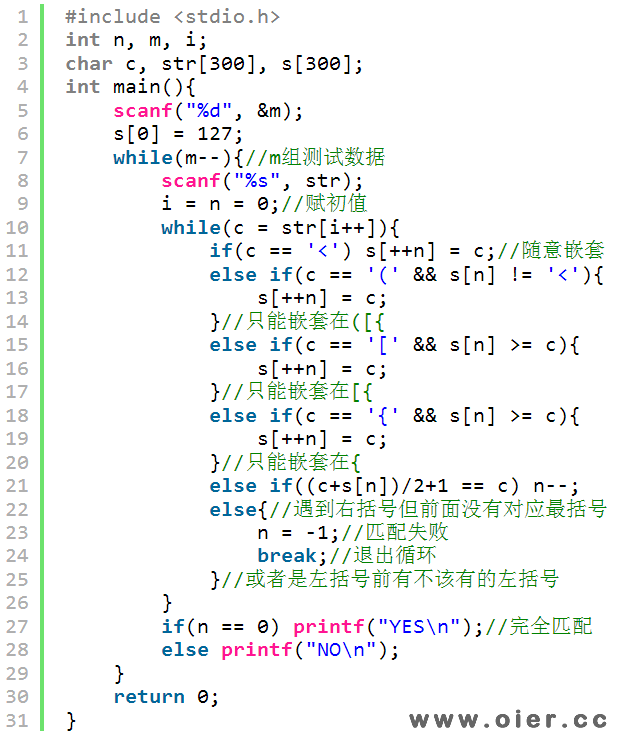SSOJ2460字符串匹配问题
1999+

## 样例输入

5
{}{}<><>()()[][]
{{}}{{}}<<>><<>>(())(())
{{}}{{}}<<>><<>>(())(())
{<>}{[]}<<<>><<>>>((<>))(())(<>)
{<>}{[]}<<<>><<>>>((<>))(())(<>)

## 样例输出

YES
YES
YES
YES
YES

# 程序实现